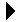Numeric constants and expressions# Numeric constants and expressionsUsing GNAT I/O Download Simple Subrange Types and Subtypes```--
-- Some numbers to come and go.
--
with Gnat.Io; use Gnat.Io;
procedure Numbers is
Mike, Alice: Integer;
John_Smith: Integer;
F: Float := 1.0;
begin
Put("Enter a number Mike: ");
Get(Mike);
Put("Enter a number Alice: ");
Get(Alice);
John_Smith := 3*Mike + 2*Alice + 2#1011#;
Put("3*Mike + 2*Alice + 11 is ");
Put(John_Smith);
New_Line;

John_Smith := Mike + Alice + 1_000_000;
Put("A million more than Mike and Alice ");
Put(John_Smith);
New_Line;

F := F + Float(Mike) + 3.14159_265;
Put("And F as an integer is ");
Put(Integer(F));
New_Line;
end Numbers;
```Using GNAT I/O Simple Subrange Types and Subtypes